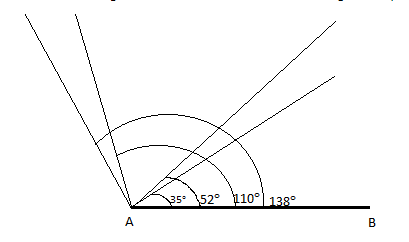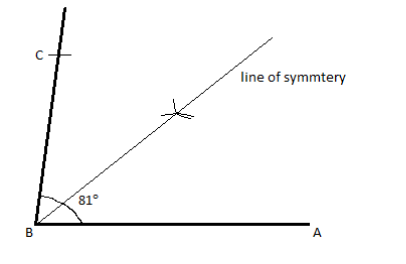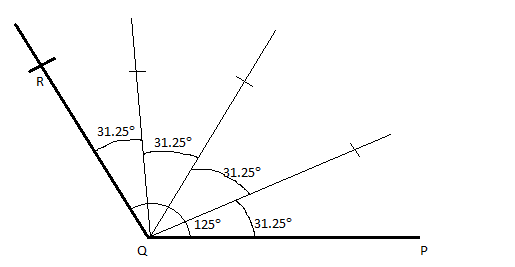QuestionAnswers

# Construct angles of given measurement with a protractor – 35°, 52°, 110° and 138°.Construct a copy of ∠ABC and ∠PQR:Draw ∠ABC = 81° and draw its line of symmetryDraw ∠PQR = 125° and divide it into 4 equal parts.

Hint: In order to make geometrical construction, we use some specific geometrical apparatus for each type of construction we make. A few geometrical apparatus are a ruler, a protractor, a pair of compasses etc. A ruler is used to measure and draw line segments, a protractor is used to measure and draw angles and a compass helps in drawing an arc or a circle.

the fixed point using a ruler, the angle subtended by these two line segments gives us the required angle.
We draw a line segment AB and measure each of the angle from point A and join them as follows:Construct a copy of ∠ABC and ∠PQR:
Given Draw ∠ABC = 81° and draw its line of symmetry
We draw a line segment AB of some length using a ruler. Then with point B as the focus we measure an angle of 81° with a protractor and mark it on the figure, then we join the line segment from B to the mark and name the point C. Thus the angle ∠ABC = 81° is obtained.
To find the line of symmetry we use a compass, with the length from B to A, we fix the stationary end of the compass on point A and draw and arc.
We repeat the same step, with the length of B to C, from point C this time.
Both these arcs intersect at a point, the line joining point B and this point of intersection is called the line of symmetry and it subtends half of the actual angle of ∠ABC on both sides of it.
It looks as follows:Given Draw ∠PQR = 125° and divide it into 4 equal parts
We draw a line segment PQ of some length using a ruler. Then with point Q as the focus we measure an angle of 125° with a protractor and mark it on the figure, then we join the line segment from B to the mark and name the point R. Thus the angle ∠PQR = 125° is obtained.
Now we are supposed to divide this angle into 4 equal parts, thus each of the divided angle should be measured.
So with respect to the line PQ we measure the angle 31.25° and mark a point, then we join the line segment from Q to this point. Then we do the same procedure for angles measuring 62.5° and 93.75° w.r.t the line PQ and join them.
Thus we end up with 4 equal halves of the angle ∠PQR measuring 31.25° each.
It is shown as follows:So, the correct answer is “Option A”.

Note: In order to solve this type of questions the key is to know how to make geometrical constructions using geometrical apparatus. We should know how to use a ruler, a compass and a protractor. We can be familiar with this by practicing more constructions. After making each construction we should check them by measuring them again to be sure. All the geometrical apparatus can be found in a geometrical box.